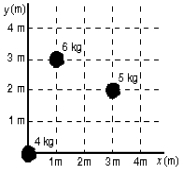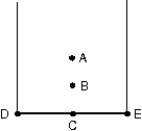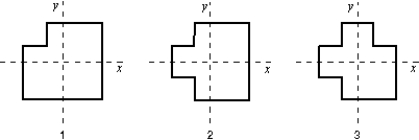# Fundamentals of Physics Study Set 2

Physics & Astronomy

## Quiz 9 :Center of Mass and Linear MomentumLooking for Introductory Physics Homework Help?

## Quiz 9 :Center of Mass and Linear Momentum

Showing 1 - 20 of 98Which one of the following statements is true?
Free
Multiple Choice

EThe x and y coordinates in meters of the center of mass of the three-particle system shown below are:Free
Multiple Choice

CThe center of mass of a uniform disk of radius R is located:
Free
Multiple Choice

EThe center of mass of the system consisting of Earth, the Sun, and the planet Mars is:
Multiple ChoiceThe center of mass of Earth's atmosphere is:
Multiple ChoiceAt the same instant that a 0.50-kg ball is dropped from 25 m above Earth, a second ball, with a mass of 0.25 kg, is thrown straight upward from Earth's surface with an initial speed of 15 m/s.They move along nearby lines and pass each other without colliding.At the end of 2.0 s the height above Earth's surface of the center of mass of the two-ball system is:
Multiple ChoiceA 640-N hunter gets a rope around a 3200-N polar bear.They are stationary, 20 m apart, on frictionless level ice.When the hunter pulls the polar bear to him, the polar bear will move:
Multiple ChoiceTwo boys with masses of 40 kg and 60 kg stand on a horizontal frictionless surface holding the ends of a light 10-m long rod.The boys pull themselves together along the rod.When they meet the 40-kg boy will have moved what distance?
Multiple ChoiceA thick uniform wire is bent into the shape of the letter "U" as shown.Which point indicates the location of the center of mass of this wire?Multiple ChoiceA machinist starts with three identical square plates but cuts one corner from one of them, two corners from the second, and three corners from the third.Rank the three plates according to the x coordinates of their centers of mass, from smallest to largest.Multiple ChoiceThe center of mass of a system of particles has a constant velocity if:
Multiple ChoiceThe center of mass of a system of particles remains at the same place if:
Multiple ChoiceA man sits in the back of a canoe in still water.He then moves to the front of the canoe and sits there.Afterwards the canoe:
Multiple ChoiceThe center of mass of a system of particles obeys an equation similar to Newton's second law where:
Multiple ChoiceA light rope passes over a light frictionless pulley attached to the ceiling.An object with a large mass is tied to one end and an object with a smaller mass is tied to the other end.Starting from rest, the heavier object moves downward, and the lighter object moves upward with an acceleration of the same magnitude.Which of the following statements is true for the system consisting of the two objects?
Multiple ChoiceTwo 4.0-kg blocks are tied together with a compressed spring between them.They are thrown from the ground with an initial velocity of 35 m/s, 45 above the horizontal.At the highest point of the trajectory they become untied and spring apart.About how far below the highest point is the center of mass of the two-block system 2.0 s later, before either fragment has hit the ground?
Multiple ChoiceBlock A, with a mass of 4.0 kg, is moving with a speed of 2.0 m/s while block B, with a mass of 8.0 kg, is moving in the opposite direction with a speed of 3.0 m/s.The center of mass of the two-block system is moving with the velocity of:
Multiple ChoiceAt the same instant that a 0.50-kg ball is dropped from 25 m above Earth, a second ball, with a mass of 0.25 kg, is thrown straight upward from Earth's surface with an initial speed of 15 m/s.They move along nearby lines and pass without colliding.At the end of 2.0 s the velocity of the center of mass of the two-ball system is:
Multiple Choice